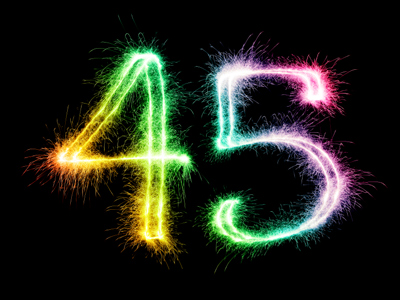If you share 45 between 5, you will get 9.

# Multiplication and Division 1

This Math quiz is called 'Multiplication and Division 1' and it has been written by teachers to help you if you are studying the subject at elementary school. Playing educational quizzes is an enjoyable way to learn if you are in the 3rd, 4th or 5th grade - aged 8 to 11.

It costs only \$12.50 per month to play this quiz and over 3,500 others that help you with your school work. You can subscribe on the page at Join Us

Multiplication and division are easier if you know your times tables. You can practice them by playing our Elementary school Times Tables Quizzes. However you still need other strategies to multiply numbers and to divide them. Some problems can be made simpler, for example to multiply 7 by 9 just multiply it by 10 and take 7 away. That makes it much easier to work out.

Take this quiz to find out how good your strategies are when multiplying or dividing numbers.

1.
What happens when a number is multiplied by 1?
The number is doubled
The number does not change
The number is halved
The digit moves one place to the right
A number does not change when it is divided by 1 either
2.
What number do I get if I share 45 between 5?
4
5
9
10
Share is another way of saying divide
3.
30 x 4 = ?
12
60
120
150
If you know 3 x 4 is 12 then just multiply by 10
4.
500 ÷ 10 = ?
5
50
250
5,000
To divide a number by 10 move the decimal point one place to the left
5.
What number do you get if you double 35?
60
70
80
90
35 x 2 = 70
6.
How many 6s in 18?
2
3
5
6
18 ÷ 6 = 3
7.
Which number sentence is incorrect?
12 x 4 = 48
4 x 12 = 48
48 ÷ 12 = 4
12 ÷ 4 = 48
12 ÷ 4 = 3 not 48!
8.
What number do you get if you double 18?
36
38
40
42
Remember that double is the same as multiply by 2
9.
How do we calculate double a number?
Multiply by 10
Divide by 2
Multiply by 2
Divide by 3
Double means the same as x 2
10.
A tower is 4 bricks tall; how many bricks in a tower 3 times taller?
1
3
12
15
4 x 3 = 12
Author:  Amanda Swift